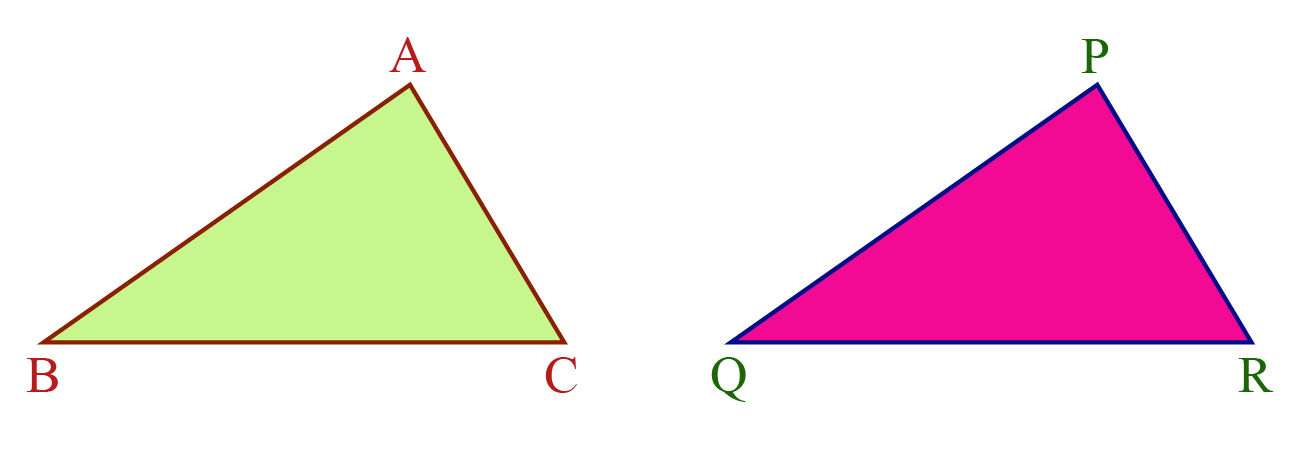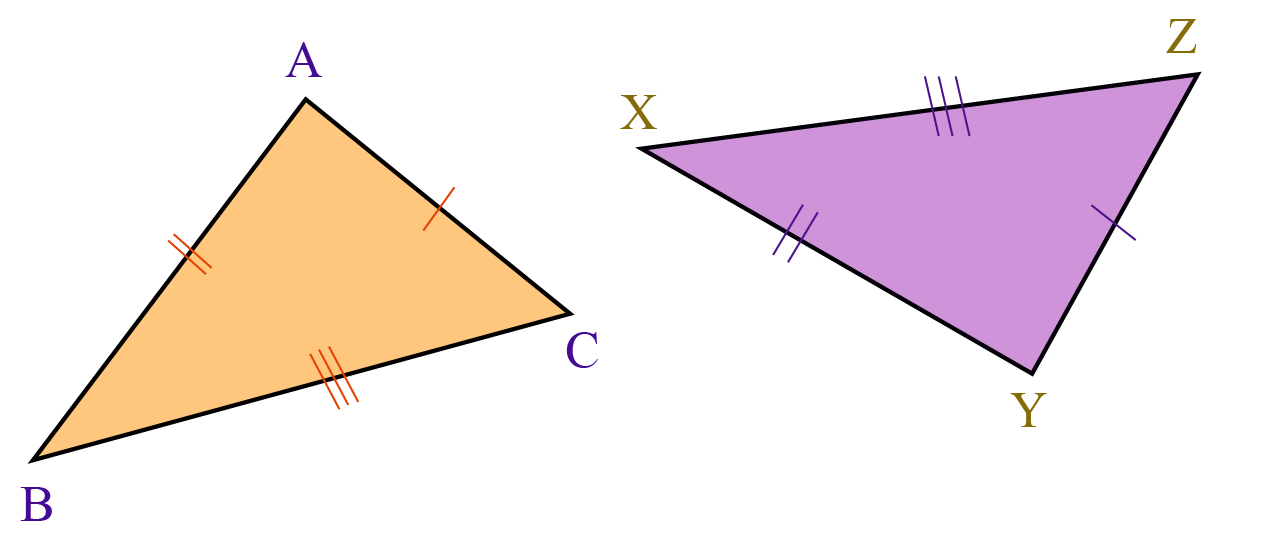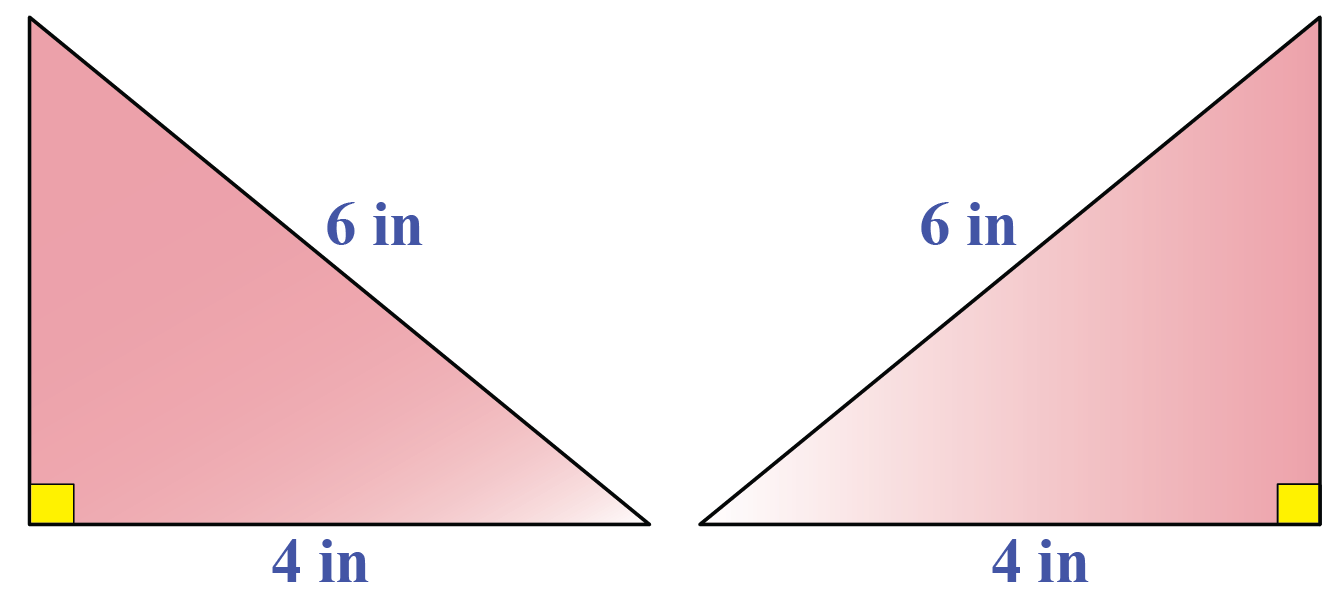# SSS

SSS

Have you ever observed that two ATM cards issued by the same bank are identical?Remember that whenever identical objects are to be produced, the concept of congruence is taken into consideration.

Congruence of triangles means that:

• All corresponding angle pairs are equal.
• All corresponding sides are proportional.

However, in order to be sure that the two triangles are similar, we do not necessarily need to have information about all sides and all angles.

In this mini-lesson, we will learn about the SSS similarity theorem in the concept of the SSS rule of congruence, using similar illustrative examples.

Check out the interactive simulation to explore more congruent shapes and do not forget to try your hand at solving a few interesting practice questions at the end of the page.

## Lesson Plan

 1 What Is the SSS Rule of Congruence? 2 Important Notes on SSS Rule of Congruence 3 Solved Examples on SSS Rule of Congruence 4 Challenging Questions on SSS Rule of Congruence 5 Interactive Questions on SSS Rule of Congruence

## What Is the SSS Rule of Congruence?

The word "congruent" means equal in every aspect or figure in terms of shape and size.

Congruence is the term used to describe the relation of two figures that are congruent.

Now let's discuss the congruence of triangles.

Look at $$\Delta ABC$$ and $$\Delta PQR$$ below.These two triangles are of the same size and shape.

Thus, we can say that they are congruent.

They can be considered as congruent triangle examples.

We can represent this in a mathematical form using the congruent triangles symbol (≅).

 $$\Delta ABC \cong \Delta PQR$$

This means $$A$$ falls on $$P$$, $$B$$ falls on $$Q$$ and $$C$$ falls on $$R$$.

Also, $$AB$$ falls on $$PQ$$, $$BC$$ falls on $$QR$$ and $$AC$$ falls on $$PR$$.

This indicates that the corresponding parts of congruent triangles are equal.

### SSS CriterionSSS Criterion stands for side side side congruence postulate.

Under this criterion, if all the three sides of one triangle are equal to the three corresponding sides of another triangle, the two triangles are congruent.

## How to Prove SSS Rule of Congruence?

Let's perform an activity to show SSS proof.

Draw two right-angled triangles with the hypotenuse of 6 inches and one side of 4 inches each.Cut these triangles and try to place one triangle over the other such that equal sides are placed over one another.

Do you observe that these two triangles superimpose on each other completely?

This means these two triangles are congruent.

This completes the SSS proof.

Do you want to explore more congruency rules?

Here is a simulation for you to explore these properties of congruent triangles.

## SSS Similarity Theorem

SSS similarity theorem says that "if we have two triangles such that the sides of one triangle are proportional to the sides of the other triangle, then these two triangles are similar to each other."

If two triangles are similar, then their corresponding angles are also equal.

grade 9 | Questions Set 1
grade 9 | Questions Set 2
More Important Topics
Numbers
Algebra
Geometry
Measurement
Money
Data
Trigonometry
Calculus
More Important Topics
Numbers
Algebra
Geometry
Measurement
Money
Data
Trigonometry
Calculus